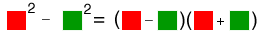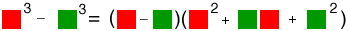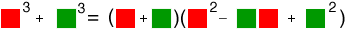Quandaries and Queries Name: larry Who is asking: Student Level: Secondary Question: how do u factor trinonmials EX: X 3 + Y 3 X 3 - 8Y 3 8X 2 - 72 64A 3 - 125B 6 Hi Larry, There are some patterns that you need to recognize in order to factor these expressions. These patterns are called the difference of squares, the difference of cubes and the sum of cubes. I see them this wayDifference of squaresDifference of cubesSum of cubes I have written them in terms of coloured patches rather than letters as often you need to see whole chunks of algebraic expressions as one patch. Your first problem is a sum of cubes where the red patch is the letter X and the green patch is the letter Y. Lets look at the second problem, X 3 - 8Y 3. There are cubes and a minus sign so my immediate thought is, can I write it as a difference of cubes? I know that 8 is 2 3 so I can write the expression as X 3 - 8Y 3 = X 3 - 2 3Y 3 = X 3 - (2Y) 3 Thus it is a difference of cubes with the red patch the letter X and the green patch 2Y. Hence, using the difference of cubes pattern I can factor this expression as X 3 - (2Y) 3 = (X - 2Y)(X 2 - X(2Y) + (2y) 2) = (X - 2Y)(X 2 - 2XY + 4y 2) For problem 3, first take out a common factor of 8. Can you see how to express the 4th problem as a difference of cubes? Penny Go to Math Central